# RD Sharma Solutions For Class 7 Maths Exercise 9.1 Chapter 9 Ratio And Proportion

Students can view and download the PDF of RD Sharma Solutions for Class 7 Maths Exercise 9.1 of Chapter 9 Ratio and Proportion are provided here. RD Sharma Solutions for Class 7 is the best study material for those who aim to secure excellent marks in their upcoming exams. The solutions are formulated by the expert team at BYJU’S in a unique manner. This exercise primarily deals with ratios of two quantities. Some of the topics are covered in this exercise are mentioned below:

• Ratio – the ratio of two quantities of the same kind and in the same units is a fraction that shows how many times the one quantity is of the other
• Ratio in simplest form

## Download the PDF of RD Sharma Solutions For Class 7 Maths Chapter 9 – Ratio and Proportion Exercise 9.1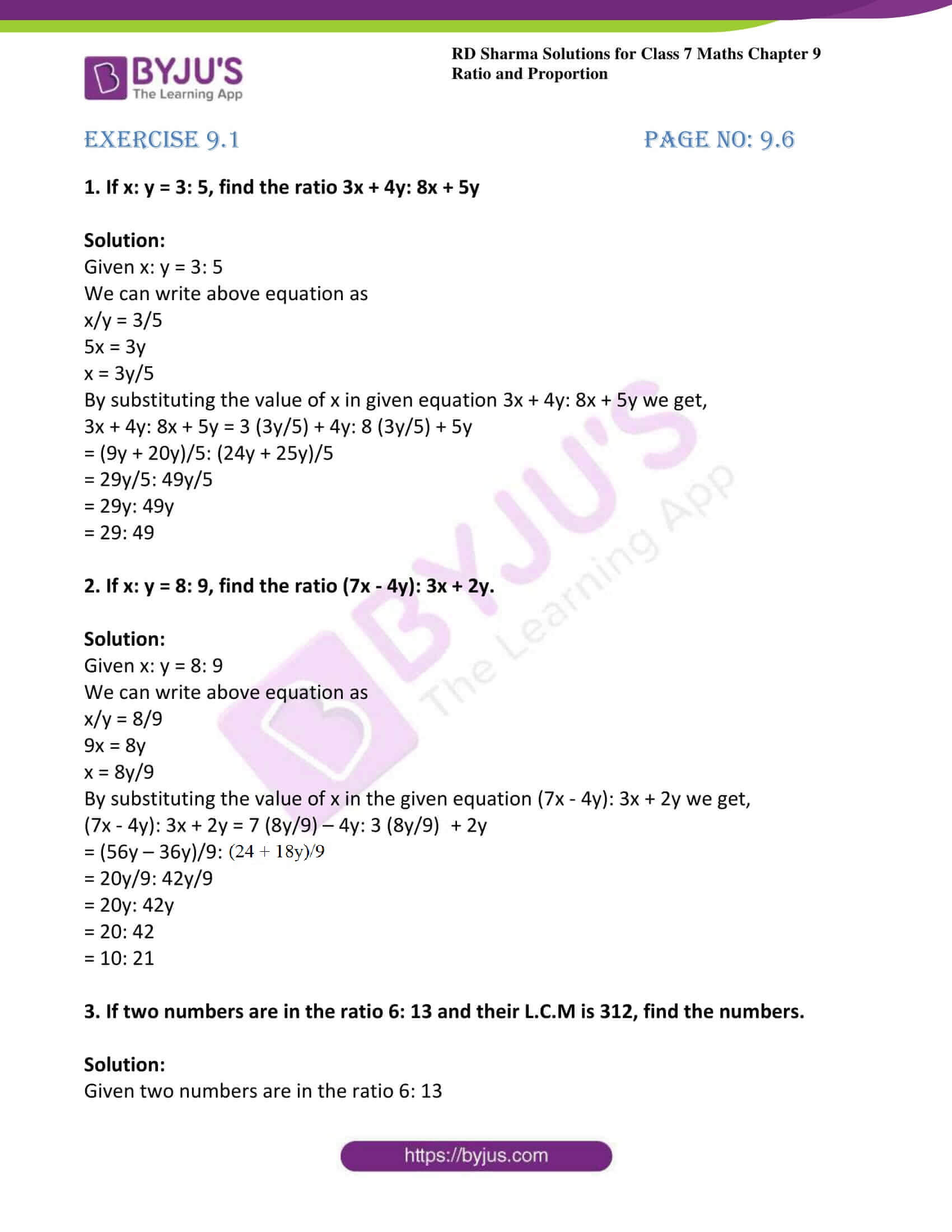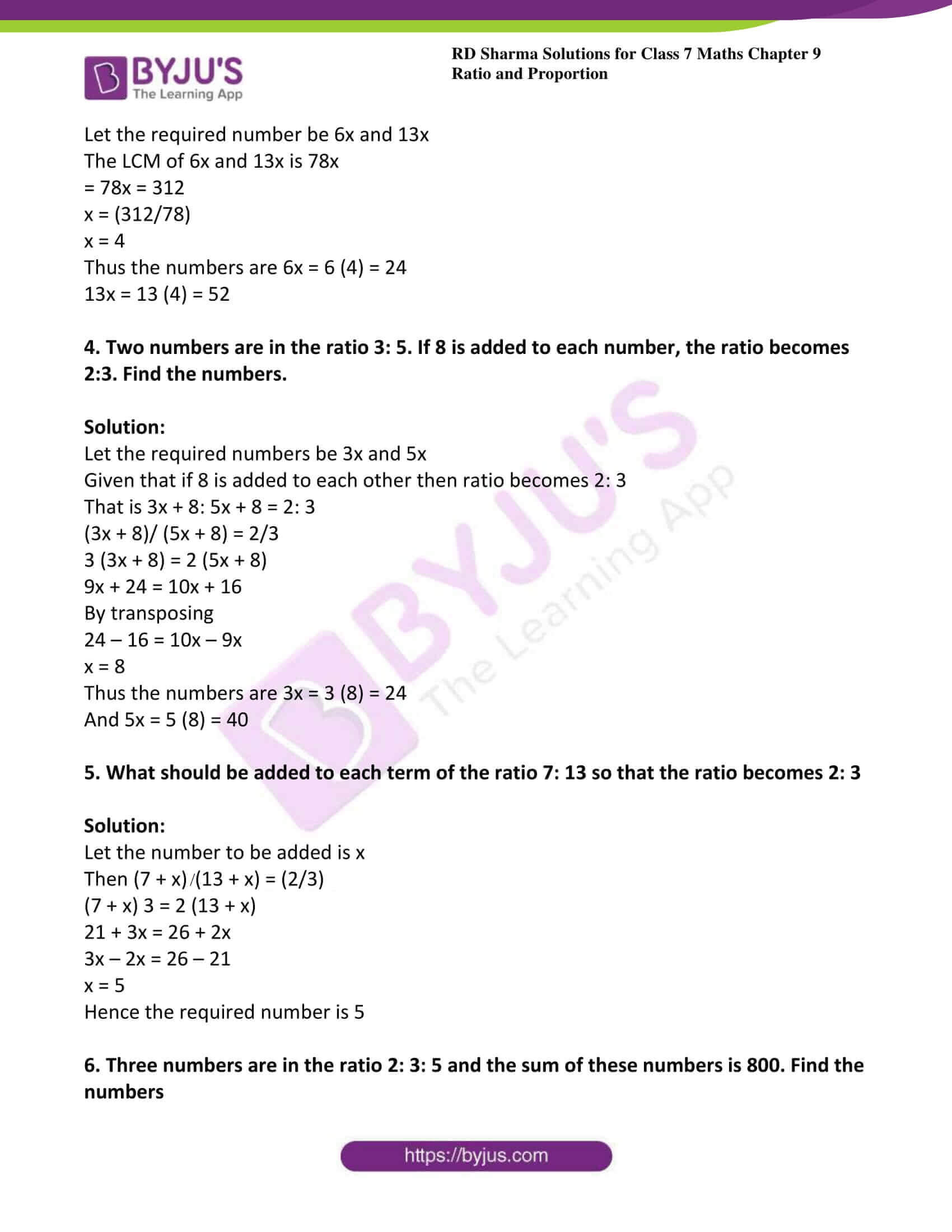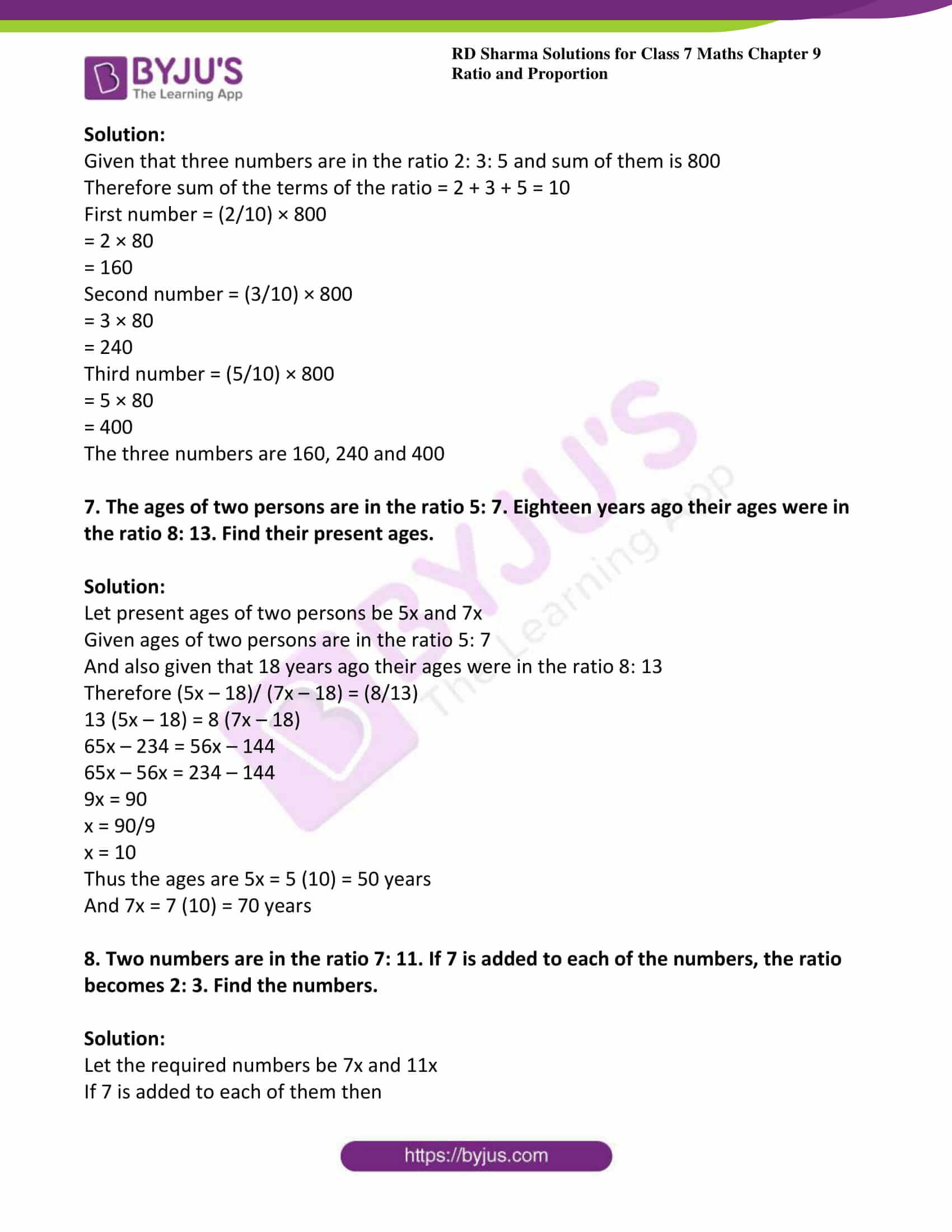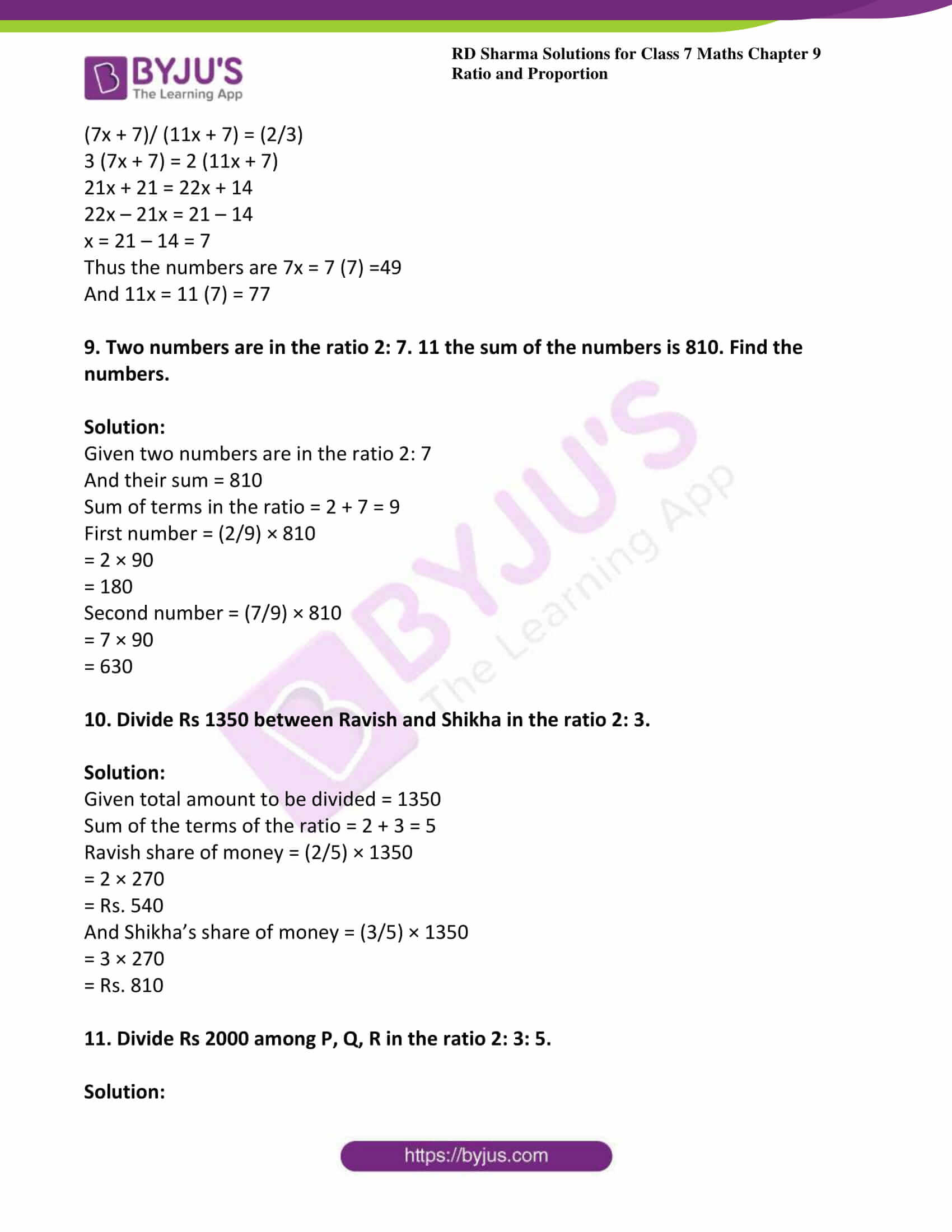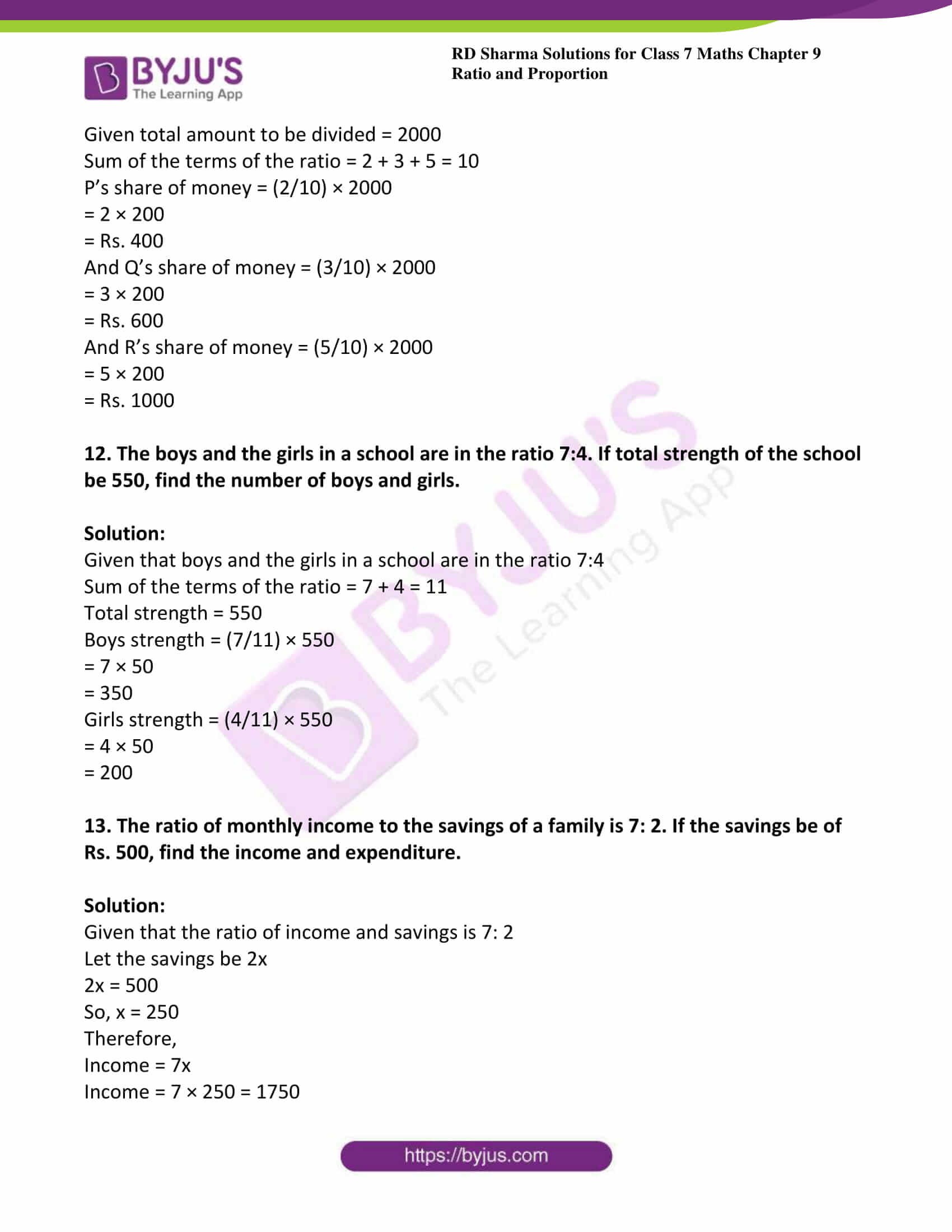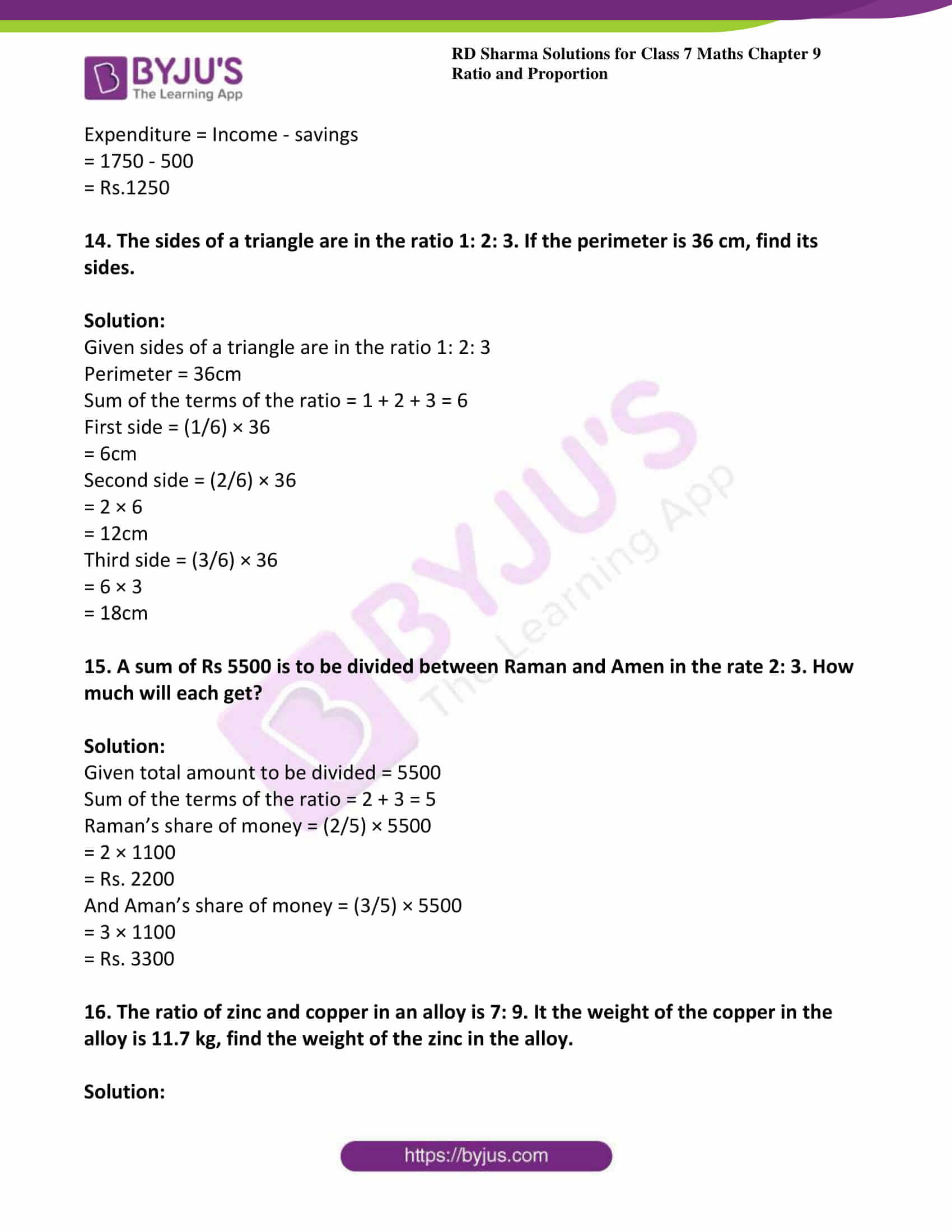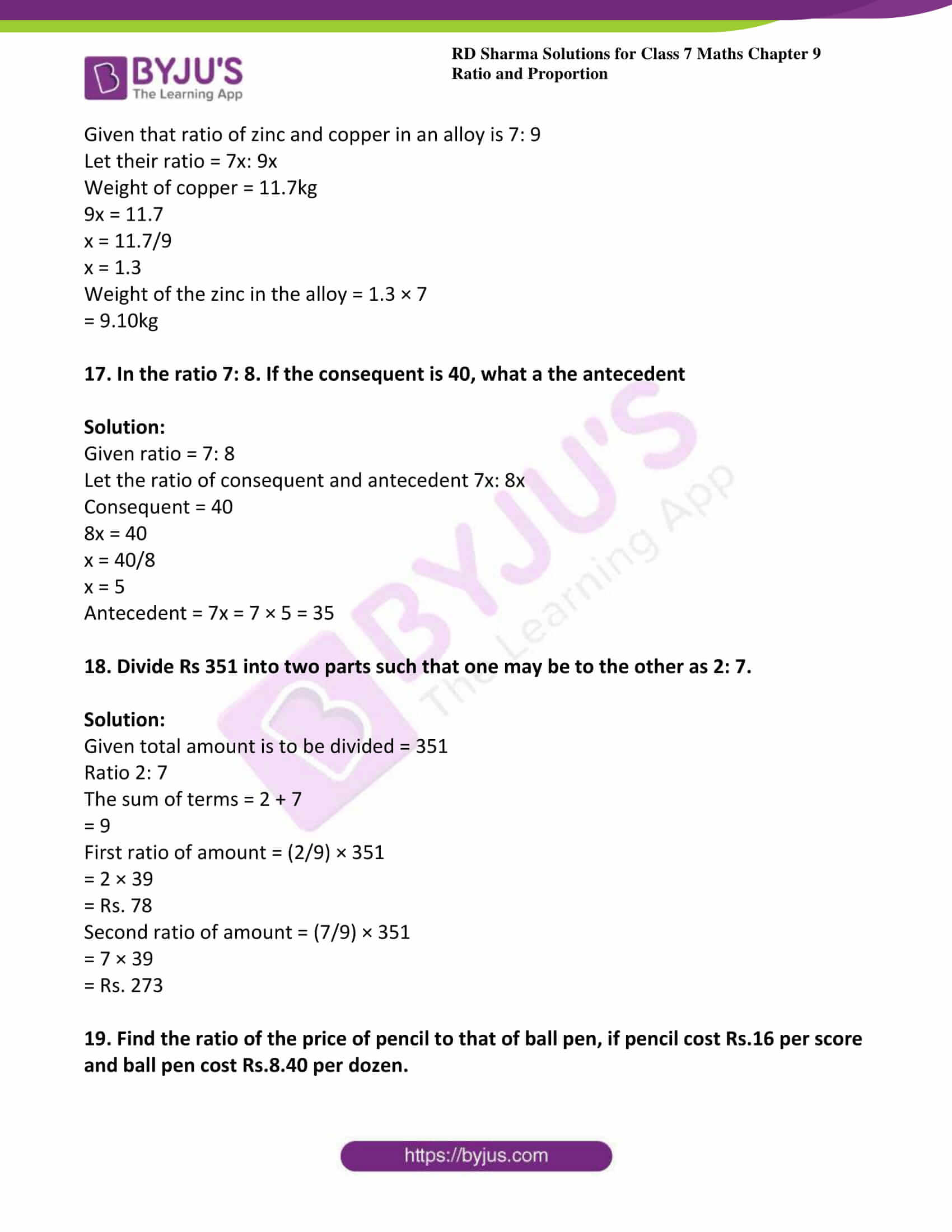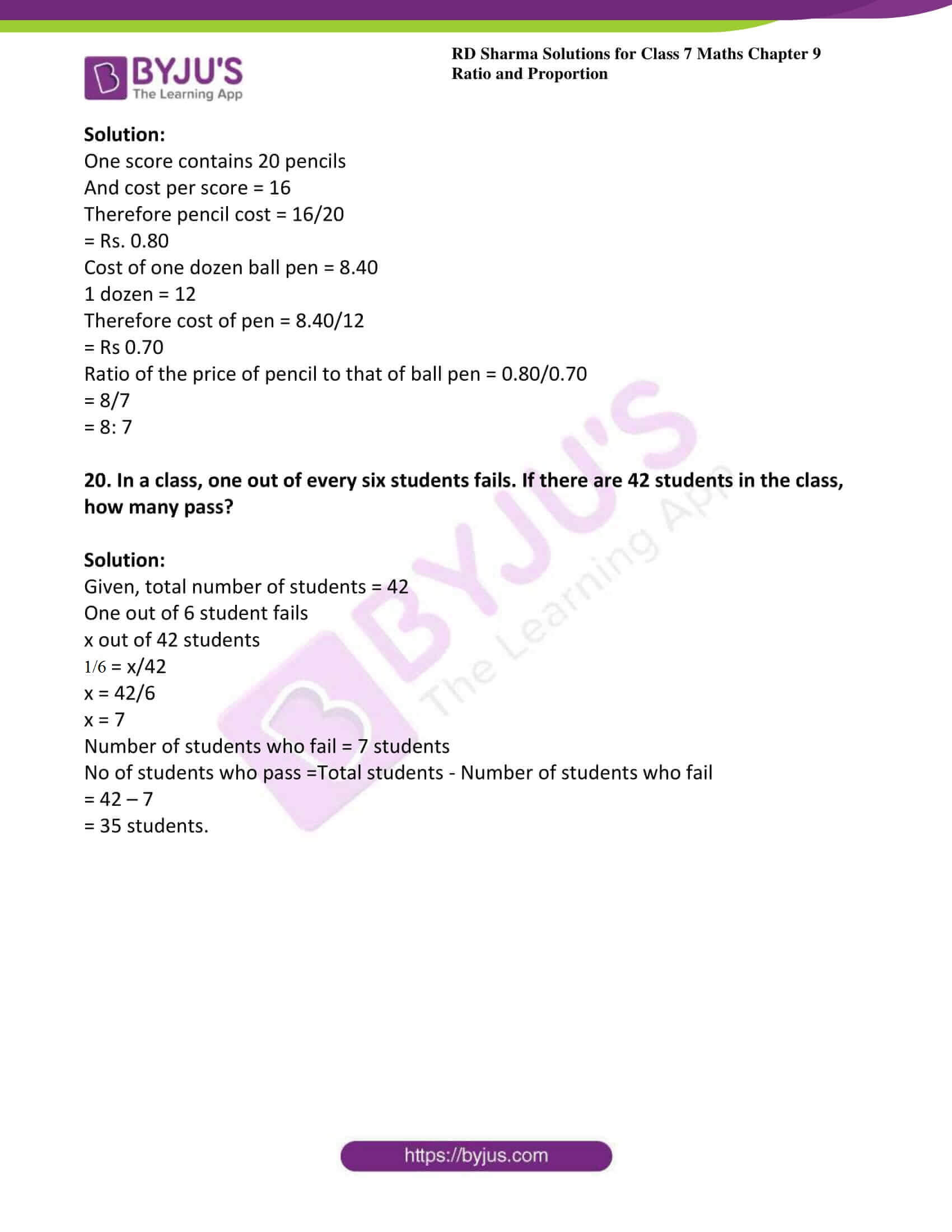### Access answers to Maths RD Sharma Solutions For Class 7 Chapter 9 – Ratio and proportion Exercise 9.1

1. If x: y = 3: 5, find the ratio 3x + 4y: 8x + 5y

Solution:

Given x: y = 3: 5

We can write above equation as

x/y = 3/5

5x = 3y

x = 3y/5

By substituting the value of x in given equation 3x + 4y: 8x + 5y we get,

3x + 4y: 8x + 5y = 3 (3y/5) + 4y: 8 (3y/5) + 5y

= (9y + 20y)/5: (24y + 25y)/5

= 29y/5: 49y/5

= 29y: 49y

= 29: 49

2. If x: y = 8: 9, find the ratio (7x – 4y): 3x + 2y.

Solution:

Given x: y = 8: 9

We can write above equation as

x/y = 8/9

9x = 8y

x = 8y/9

By substituting the value of x in the given equation (7x – 4y): 3x + 2y we get,

(7x – 4y): 3x + 2y = 7 (8y/9) – 4y: 3 (8y/9) + 2y

= (56y – 36y)/9: (24y + 18y)/9

= 20y/9: 42y/9

= 20y: 42y

= 20: 42

= 10: 21

3. If two numbers are in the ratio 6: 13 and their L.C.M is 312, find the numbers.

Solution:

Given two numbers are in the ratio 6: 13

Let the required number be 6x and 13x

The LCM of 6x and 13x is 78x

= 78x = 312

x = (312/78)

x = 4

Thus the numbers are 6x = 6 (4) = 24

13x = 13 (4) = 52

4. Two numbers are in the ratio 3: 5. If 8 is added to each number, the ratio becomes 2:3. Find the numbers.

Solution:

Let the required numbers be 3x and 5x

Given that if 8 is added to each other then ratio becomes 2: 3

That is 3x + 8: 5x + 8 = 2: 3

(3x + 8)/ (5x + 8) = 2/3

3 (3x + 8) = 2 (5x + 8)

9x + 24 = 10x + 16

By transposing

24 – 16 = 10x – 9x

x = 8

Thus the numbers are 3x = 3 (8) = 24

And 5x = 5 (8) = 40

5. What should be added to each term of the ratio 7: 13 so that the ratio becomes 2: 3

Solution:

Let the number to be added is x

Then (7 + x)/ (13 + x) = (2/3)

(7 + x) 3 = 2 (13 + x)

21 + 3x = 26 + 2x

3x – 2x = 26 – 21

x = 5

Hence the required number is 5

6. Three numbers are in the ratio 2: 3: 5 and the sum of these numbers is 800. Find the numbers

Solution:

Given that three numbers are in the ratio 2: 3: 5 and sum of them is 800

Therefore sum of the terms of the ratio = 2 + 3 + 5 = 10

First number = (2/10) × 800

= 2 × 80

= 160

Second number = (3/10) × 800

= 3 × 80

= 240

Third number = (5/10) × 800

= 5 × 80

= 400

The three numbers are 160, 240 and 400

7. The ages of two persons are in the ratio 5: 7. Eighteen years ago their ages were in the ratio 8: 13. Find their present ages.

Solution:

Let present ages of two persons be 5x and 7x

Given ages of two persons are in the ratio 5: 7

And also given that 18 years ago their ages were in the ratio 8: 13

Therefore (5x – 18)/ (7x – 18) = (8/13)

13 (5x – 18) = 8 (7x – 18)

65x – 234 = 56x – 144

65x – 56x = 234 – 144

9x = 90

x = 90/9

x = 10

Thus the ages are 5x = 5 (10) = 50 years

And 7x = 7 (10) = 70 years

8. Two numbers are in the ratio 7: 11. If 7 is added to each of the numbers, the ratio becomes 2: 3. Find the numbers.

Solution:

Let the required numbers be 7x and 11x

If 7 is added to each of them then

(7x + 7)/ (11x + 7) = (2/3)

3 (7x + 7) = 2 (11x + 7)

21x + 21 = 22x + 14

22x – 21x = 21 – 14

x = 21 – 14 = 7

Thus the numbers are 7x = 7 (7) =49

And 11x = 11 (7) = 77

9. Two numbers are in the ratio 2: 7. 11 the sum of the numbers is 810. Find the numbers.

Solution:

Given two numbers are in the ratio 2: 7

And their sum = 810

Sum of terms in the ratio = 2 + 7 = 9

First number = (2/9) × 810

= 2 × 90

= 180

Second number = (7/9) × 810

= 7 × 90

= 630

10. Divide Rs 1350 between Ravish and Shikha in the ratio 2: 3.

Solution:

Given total amount to be divided = 1350

Sum of the terms of the ratio = 2 + 3 = 5

Ravish share of money = (2/5) × 1350

= 2 × 270

= Rs. 540

And Shikha’s share of money = (3/5) × 1350

= 3 × 270

= Rs. 810

11. Divide Rs 2000 among P, Q, R in the ratio 2: 3: 5.

Solution:

Given total amount to be divided = 2000

Sum of the terms of the ratio = 2 + 3 + 5 = 10

P’s share of money = (2/10) × 2000

= 2 × 200

= Rs. 400

And Q’s share of money = (3/10) × 2000

= 3 × 200

= Rs. 600

And R’s share of money = (5/10) × 2000

= 5 × 200

= Rs. 1000

12. The boys and the girls in a school are in the ratio 7:4. If total strength of the school be 550, find the number of boys and girls.

Solution:

Given that boys and the girls in a school are in the ratio 7:4

Sum of the terms of the ratio = 7 + 4 = 11

Total strength = 550

Boys strength = (7/11) × 550

= 7 × 50

= 350

Girls strength = (4/11) × 550

= 4 × 50

= 200

13. The ratio of monthly income to the savings of a family is 7: 2. If the savings be of Rs. 500, find the income and expenditure.

Solution:

Given that the ratio of income and savings is 7: 2

Let the savings be 2x

2x = 500

So, x = 250

Therefore,

Income = 7x

Income = 7 × 250 = 1750

Expenditure = Income – savings

= 1750 – 500

= Rs.1250

14. The sides of a triangle are in the ratio 1: 2: 3. If the perimeter is 36 cm, find its sides.

Solution:

Given sides of a triangle are in the ratio 1: 2: 3

Perimeter = 36cm

Sum of the terms of the ratio = 1 + 2 + 3 = 6

First side = (1/6) × 36

= 6cm

Second side = (2/6) × 36

= 2 × 6

= 12cm

Third side = (3/6) × 36

= 6 × 3

= 18cm

15. A sum of Rs 5500 is to be divided between Raman and Amen in the rate 2: 3. How much will each get?

Solution:

Given total amount to be divided = 5500

Sum of the terms of the ratio = 2 + 3 = 5

Raman’s share of money = (2/5) × 5500

= 2 × 1100

= Rs. 2200

And Aman’s share of money = (3/5) × 5500

= 3 × 1100

= Rs. 3300

16. The ratio of zinc and copper in an alloy is 7: 9. It the weight of the copper in the alloy is 11.7 kg, find the weight of the zinc in the alloy.

Solution:

Given that ratio of zinc and copper in an alloy is 7: 9

Let their ratio = 7x: 9x

Weight of copper = 11.7kg

9x = 11.7

x = 11.7/9

x = 1.3

Weight of the zinc in the alloy = 1.3 × 7

= 9.10kg

17. In the ratio 7: 8. If the consequent is 40, what a the antecedent

Solution:

Given ratio = 7: 8

Let the ratio of consequent and antecedent 7x: 8x

Consequent = 40

8x = 40

x = 40/8

x = 5

Antecedent = 7x = 7 × 5 = 35

18. Divide Rs 351 into two parts such that one may be to the other as 2: 7.

Solution:

Given total amount is to be divided = 351

Ratio 2: 7

The sum of terms = 2 + 7

= 9

First ratio of amount = (2/9) × 351

= 2 × 39

= Rs. 78

Second ratio of amount = (7/9) × 351

= 7 × 39

= Rs. 273

19. Find the ratio of the price of pencil to that of ball pen, if pencil cost Rs.16 per score and ball pen cost Rs.8.40 per dozen.

Solution:

One score contains 20 pencils

And cost per score = 16

Therefore pencil cost = 16/20

= Rs. 0.80

Cost of one dozen ball pen = 8.40

1 dozen = 12

Therefore cost of pen = 8.40/12

= Rs 0.70

Ratio of the price of pencil to that of ball pen = 0.80/0.70

= 8/7

= 8: 7

20. In a class, one out of every six students fails. If there are 42 students in the class, how many pass?

Solution:

Given, total number of students = 42

One out of 6 student fails

x out of 42 students

1/6 = x/42

x = 42/6

x = 7

Number of students who fail = 7 students

No of students who pass =Total students – Number of students who fail

= 42 – 7

= 35 students.